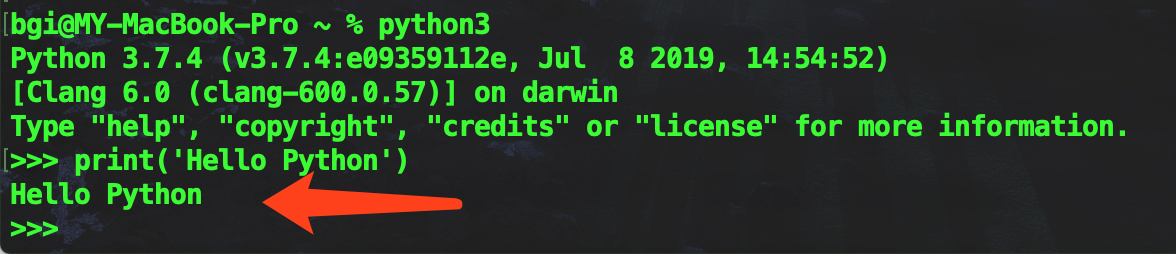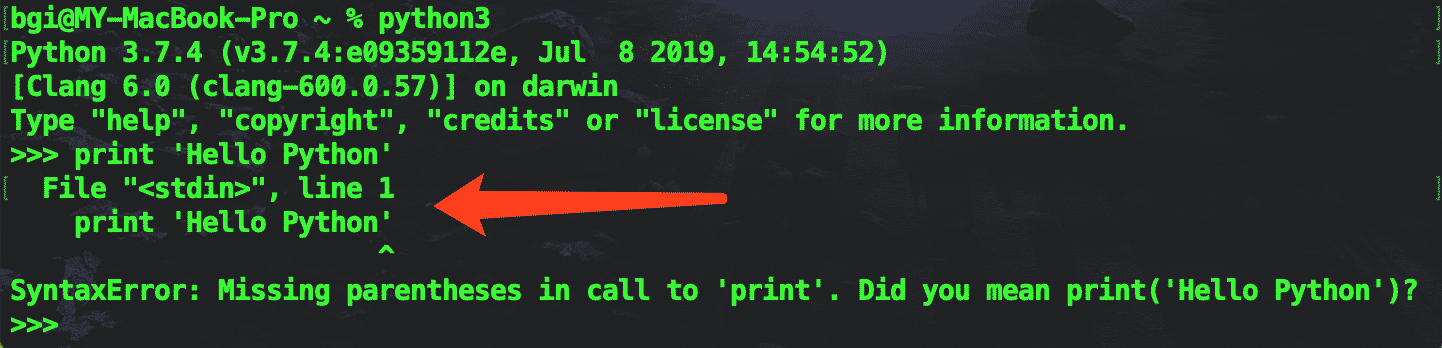## Python3报错• 语法错误
• 逻辑异常

### 语法错误

``````list = ['苹果', '西瓜', '葡萄']
for lt in list
print(lt)

for lt in list
^
SyntaxError: invalid syntax``````

``````list = ['苹果', '西瓜', '葡萄']
for lt in list:
print(lt)

print(lt)
^
IndentationError: expected an indented block``````

### 逻辑异常

``````a = 1 / 0
print(a)

a = 1 / 0
ZeroDivisionError: division by zero``````

``````a = 1 / b
print(a)

a = 1 / b
NameError: name 'b' is not defined``````

## 异常处理

``````try:
1/0
except:
print('程序报错了')

print('无论1/0对不对，我都不希望程序中止')``````

``````try:
a/0
except ZeroDivisionError:
print('0不能作为被除数')
except NameError:
print('变量没有被定义')``````

``````try:
a/0
except ZeroDivisionError:
print('0不能作为被除数')
except NameError:
print('变量没有被定义')
else:
print('没有任何报错')``````

### 练习题

``````x = int(input("请输入一个整数: "))

y = 1 / x
print(x + "的倒数是：" + y)``````### 习题答案

``````try:
x = int(input("请输入一个整数: "))

y = 1 / x
print(x + "的倒数是：" + y)
except ZeroDivisionError:
print('0不能作为被除数')
except NameError:
print('变量没有被定义')``````